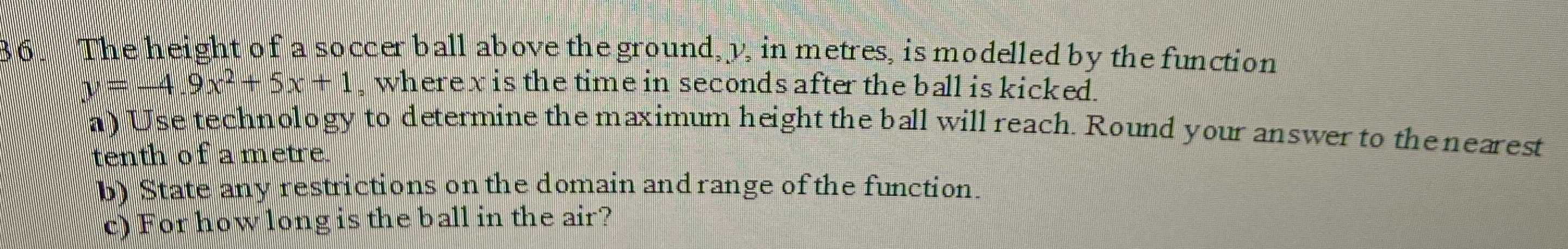### ¿Todavía tienes preguntas de matemáticas?

Pregunte a nuestros tutores expertos
Algebra
Pregunta36. The height of a soccer ball above the ground, $$y$$ , in metres, is modelled by the function

$$y = - 4.9 x ^ { 2 } + 5 x + 1$$ , where $$x$$ is the time in seconds after the ball is kicked.

a) Use technology to determine the maximum height the ball will reach. Round your answer to the nearest tenth of a metre.

b) State any restrictions on the domain and range of the function.

c) For how long is the ball in the air?

b) domain: x$$\ge$$0
range: y$$\ge$$0